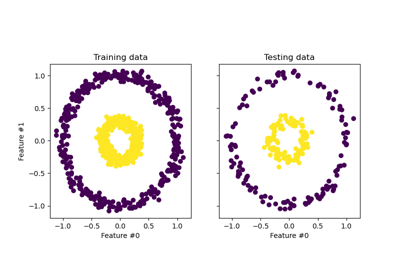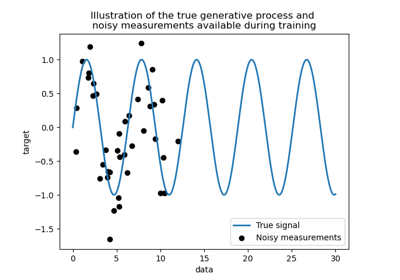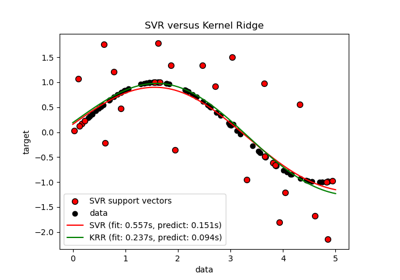# sklearn.kernel_ridge.KernelRidge¶

class sklearn.kernel_ridge.KernelRidge(alpha=1, *, kernel='linear', gamma=None, degree=3, coef0=1, kernel_params=None)[source]

Kernel ridge regression.

Kernel ridge regression (KRR) combines ridge regression (linear least squares with l2-norm regularization) with the kernel trick. It thus learns a linear function in the space induced by the respective kernel and the data. For non-linear kernels, this corresponds to a non-linear function in the original space.

The form of the model learned by KRR is identical to support vector regression (SVR). However, different loss functions are used: KRR uses squared error loss while support vector regression uses epsilon-insensitive loss, both combined with l2 regularization. In contrast to SVR, fitting a KRR model can be done in closed-form and is typically faster for medium-sized datasets. On the other hand, the learned model is non-sparse and thus slower than SVR, which learns a sparse model for epsilon > 0, at prediction-time.

This estimator has built-in support for multi-variate regression (i.e., when y is a 2d-array of shape [n_samples, n_targets]).

Read more in the User Guide.

Parameters:
alphafloat or array-like of shape (n_targets,), default=1.0

Regularization strength; must be a positive float. Regularization improves the conditioning of the problem and reduces the variance of the estimates. Larger values specify stronger regularization. Alpha corresponds to 1 / (2C) in other linear models such as LogisticRegression or LinearSVC. If an array is passed, penalties are assumed to be specific to the targets. Hence they must correspond in number. See Ridge regression and classification for formula.

kernelstr or callable, default=”linear”

Kernel mapping used internally. This parameter is directly passed to pairwise_kernels. If kernel is a string, it must be one of the metrics in pairwise.PAIRWISE_KERNEL_FUNCTIONS or “precomputed”. If kernel is “precomputed”, X is assumed to be a kernel matrix. Alternatively, if kernel is a callable function, it is called on each pair of instances (rows) and the resulting value recorded. The callable should take two rows from X as input and return the corresponding kernel value as a single number. This means that callables from sklearn.metrics.pairwise are not allowed, as they operate on matrices, not single samples. Use the string identifying the kernel instead.

gammafloat, default=None

Gamma parameter for the RBF, laplacian, polynomial, exponential chi2 and sigmoid kernels. Interpretation of the default value is left to the kernel; see the documentation for sklearn.metrics.pairwise. Ignored by other kernels.

degreeint, default=3

Degree of the polynomial kernel. Ignored by other kernels.

coef0float, default=1

Zero coefficient for polynomial and sigmoid kernels. Ignored by other kernels.

kernel_paramsdict, default=None

Additional parameters (keyword arguments) for kernel function passed as callable object.

Attributes:
dual_coef_ndarray of shape (n_samples,) or (n_samples, n_targets)

Representation of weight vector(s) in kernel space

X_fit_{ndarray, sparse matrix} of shape (n_samples, n_features)

Training data, which is also required for prediction. If kernel == “precomputed” this is instead the precomputed training matrix, of shape (n_samples, n_samples).

n_features_in_int

Number of features seen during fit.

New in version 0.24.

feature_names_in_ndarray of shape (n_features_in_,)

Names of features seen during fit. Defined only when X has feature names that are all strings.

New in version 1.0.

sklearn.gaussian_process.GaussianProcessRegressor

Gaussian Process regressor providing automatic kernel hyperparameters tuning and predictions uncertainty.

sklearn.linear_model.Ridge

Linear ridge regression.

sklearn.linear_model.RidgeCV

Ridge regression with built-in cross-validation.

sklearn.svm.SVR

Support Vector Regression accepting a large variety of kernels.

References

• Kevin P. Murphy “Machine Learning: A Probabilistic Perspective”, The MIT Press chapter 14.4.3, pp. 492-493

Examples

>>> from sklearn.kernel_ridge import KernelRidge
>>> import numpy as np
>>> n_samples, n_features = 10, 5
>>> rng = np.random.RandomState(0)
>>> y = rng.randn(n_samples)
>>> X = rng.randn(n_samples, n_features)
>>> krr = KernelRidge(alpha=1.0)
>>> krr.fit(X, y)
KernelRidge(alpha=1.0)


Methods

 fit(X, y[, sample_weight]) Fit Kernel Ridge regression model. Get metadata routing of this object. get_params([deep]) Get parameters for this estimator. Predict using the kernel ridge model. score(X, y[, sample_weight]) Return the coefficient of determination of the prediction. set_fit_request(*[, sample_weight]) Request metadata passed to the fit method. set_params(**params) Set the parameters of this estimator. set_score_request(*[, sample_weight]) Request metadata passed to the score method.
fit(X, y, sample_weight=None)[source]

Fit Kernel Ridge regression model.

Parameters:
X{array-like, sparse matrix} of shape (n_samples, n_features)

Training data. If kernel == “precomputed” this is instead a precomputed kernel matrix, of shape (n_samples, n_samples).

yarray-like of shape (n_samples,) or (n_samples, n_targets)

Target values.

sample_weightfloat or array-like of shape (n_samples,), default=None

Individual weights for each sample, ignored if None is passed.

Returns:
selfobject

Returns the instance itself.

Get metadata routing of this object.

Please check User Guide on how the routing mechanism works.

Returns:

A MetadataRequest encapsulating routing information.

get_params(deep=True)[source]

Get parameters for this estimator.

Parameters:
deepbool, default=True

If True, will return the parameters for this estimator and contained subobjects that are estimators.

Returns:
paramsdict

Parameter names mapped to their values.

predict(X)[source]

Predict using the kernel ridge model.

Parameters:
X{array-like, sparse matrix} of shape (n_samples, n_features)

Samples. If kernel == “precomputed” this is instead a precomputed kernel matrix, shape = [n_samples, n_samples_fitted], where n_samples_fitted is the number of samples used in the fitting for this estimator.

Returns:
Cndarray of shape (n_samples,) or (n_samples, n_targets)

Returns predicted values.

score(X, y, sample_weight=None)[source]

Return the coefficient of determination of the prediction.

The coefficient of determination $$R^2$$ is defined as $$(1 - \frac{u}{v})$$, where $$u$$ is the residual sum of squares ((y_true - y_pred)** 2).sum() and $$v$$ is the total sum of squares ((y_true - y_true.mean()) ** 2).sum(). The best possible score is 1.0 and it can be negative (because the model can be arbitrarily worse). A constant model that always predicts the expected value of y, disregarding the input features, would get a $$R^2$$ score of 0.0.

Parameters:
Xarray-like of shape (n_samples, n_features)

Test samples. For some estimators this may be a precomputed kernel matrix or a list of generic objects instead with shape (n_samples, n_samples_fitted), where n_samples_fitted is the number of samples used in the fitting for the estimator.

yarray-like of shape (n_samples,) or (n_samples, n_outputs)

True values for X.

sample_weightarray-like of shape (n_samples,), default=None

Sample weights.

Returns:
scorefloat

$$R^2$$ of self.predict(X) w.r.t. y.

Notes

The $$R^2$$ score used when calling score on a regressor uses multioutput='uniform_average' from version 0.23 to keep consistent with default value of r2_score. This influences the score method of all the multioutput regressors (except for MultiOutputRegressor).

set_fit_request(*, sample_weight: Union[bool, None, str] = '$UNCHANGED$') [source]

Request metadata passed to the fit method.

Note that this method is only relevant if enable_metadata_routing=True (see sklearn.set_config). Please see User Guide on how the routing mechanism works.

The options for each parameter are:

• True: metadata is requested, and passed to fit if provided. The request is ignored if metadata is not provided.

• False: metadata is not requested and the meta-estimator will not pass it to fit.

• None: metadata is not requested, and the meta-estimator will raise an error if the user provides it.

• str: metadata should be passed to the meta-estimator with this given alias instead of the original name.

The default (sklearn.utils.metadata_routing.UNCHANGED) retains the existing request. This allows you to change the request for some parameters and not others.

New in version 1.3.

Note

This method is only relevant if this estimator is used as a sub-estimator of a meta-estimator, e.g. used inside a Pipeline. Otherwise it has no effect.

Parameters:
sample_weightstr, True, False, or None, default=sklearn.utils.metadata_routing.UNCHANGED

Metadata routing for sample_weight parameter in fit.

Returns:
selfobject

The updated object.

set_params(**params)[source]

Set the parameters of this estimator.

The method works on simple estimators as well as on nested objects (such as Pipeline). The latter have parameters of the form <component>__<parameter> so that it’s possible to update each component of a nested object.

Parameters:
**paramsdict

Estimator parameters.

Returns:
selfestimator instance

Estimator instance.

set_score_request(*, sample_weight: Union[bool, None, str] = '$UNCHANGED$') [source]

Request metadata passed to the score method.

Note that this method is only relevant if enable_metadata_routing=True (see sklearn.set_config). Please see User Guide on how the routing mechanism works.

The options for each parameter are:

• True: metadata is requested, and passed to score if provided. The request is ignored if metadata is not provided.

• False: metadata is not requested and the meta-estimator will not pass it to score.

• None: metadata is not requested, and the meta-estimator will raise an error if the user provides it.

• str: metadata should be passed to the meta-estimator with this given alias instead of the original name.

The default (sklearn.utils.metadata_routing.UNCHANGED) retains the existing request. This allows you to change the request for some parameters and not others.

New in version 1.3.

Note

This method is only relevant if this estimator is used as a sub-estimator of a meta-estimator, e.g. used inside a Pipeline. Otherwise it has no effect.

Parameters:
sample_weightstr, True, False, or None, default=sklearn.utils.metadata_routing.UNCHANGED

Metadata routing for sample_weight parameter in score.

Returns:
selfobject

The updated object.

## Examples using sklearn.kernel_ridge.KernelRidge¶Kernel PCA

Kernel PCAComparison of kernel ridge and Gaussian process regression

Comparison of kernel ridge and Gaussian process regressionComparison of kernel ridge regression and SVR

Comparison of kernel ridge regression and SVR